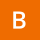Home Communities
IT Knowledge
Inspiration
Languages
EN

# JavaScript - calculate sin in degrees

7 points
Created by:Blessing-D
574

In this article, we would like to show you a simple example of how to calculate sin in degrees in JavaScript.

## Practical example

In order to use this example we just need to change the value of `deg` variable to some other value (in degrees).

``````// ONLINE-RUNNER:browser;

function calculateSin(deg) {
var radians = (Math.PI / 180) * deg;

}

var deg = 30;
var y = calculateSin(deg);

// sin(30 deg) = 0.49999999999999994
console.log('sin(' + deg + ' deg) = ' + y);``````

Output:

``sin(30 deg) = 0.49999999999999994``

We can change the value of `deg`.

Example of common calculations:

``````sin(0 deg) = 0
sin(15 deg) = 0.25881904510252074
sin(30 deg) = 0.49999999999999994
sin(45 deg) = 0.7071067811865475
sin(60 deg) = 0.8660254037844386
sin(90 deg) = 1``````

I've prepared a similar post but for calculation cos in degrees:

Join to our subscribers to be up to date with content, news and offers.